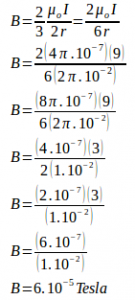# Magnetic field at the center of an arc of current – problems and solutions

1. Based on the figure below, if the radius of the curvature of the wire is 50 cm, determine the magnitude of the magnetic field at the center of curvature (at point 0, see figure below). (µo = 4π.10-7 Wb.A-1 m-1)

Known :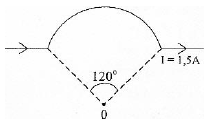Radius (r) = 50 cm = 0.5 m

Electric current (I) = 1.5 Ampere

The vacuum permeability o) = 4π.10-7 Wb.A-1 m-1

Wanted: The magnitude of the magnetic field

Solution :

360o = 1 circumference of a circle. 120o / 360o = 1/3 then 120o = 1/3 x circumference of a circle.

The equation of the magnetic field at the center of the coil with several loops :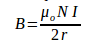B = the magnitude of the magnetic field, N = number of loops, I = electric current, r = radius of curvature

In the above problem, there is only one loop so that N is eliminated from the equation. The wire coil on the above problem is not 1 circle but 1/3 circle :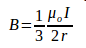The magnitude of the magnetic field at the center of curvature :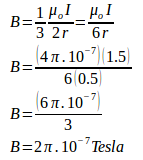2. Based on the figure below, the electric current flows in the wire is 6-A and radius of curvature is R = 3π cm, to determine the magnitude of the magnetic field at point P.

Known :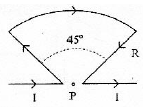Radius of curvature (r) = 3π cm = (3π/100) m

= 3π/102 m = 3π.10-2 m

Electric current (I) = 6 A

The vacuum permeability o) = 4π.10-7 Wb.A-1 m-1

Wanted : The magnitude of the magnetic field

Solution :

360o = 1 circumference of a circle. 45o / 360o = 1/8 then 45o = 1/8 x circumference of a circle.

The magnitude of the magnetic field at the center of curvature :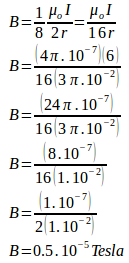3. Electric current flows in wire = 9-A, the radius of curvature (R) = 2π cm and µo = 4π.10-7 Wb.A-1.m-1, determine the magnitude of the magnetic field at point P.

Known :

Radius of curvature (r) = 2π cm = (2π/100) m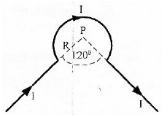= 2π/102 m = 2π.10-2 m

Electric current (I) = 9 A

The vacuum permeability o) = 4π.10-7 Wb.A-1 m-1

Wanted : The magnitude of the magnetic field at point P

Solution :

360o – 120o = 240o. 240o / 360o = 2/3 then 240o = 2/3 x circumference of a circle.

The magnitude of the magnetic field at the center of curvature :